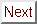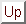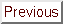Next: Molecular surface and volume Up: Molecular Modeling Previous: Computer representation of chemical

# Computer representation of geometry

Molecules are not static entities. Even at absolute zero temperature atoms in a molecule are actively vibrating. The molecular geometry represented by a static picture on the computer screen or a Dreiding model is therefore only an approximation. The term atom position is usually understood as a position of the atom nucleus, or rather as some kind of average position of the vibrating nucleus. Luckily, the dimensions of an atom nucleus are negligible compared to average bond lengths, and since its mass is thousands of times larger than the mass of surrounding electrons, the nucleus is the true center of gravity of an atom. The major conceptual difficulty is to decide what is an average position of a nucleus. Nuclear vibrations are anharmonic, and hence, the time average position of a nucleus is not located half way between its extreme positions. Moreover, in molecules containing more than two atoms, nuclei vibrate not only along chemical bonds but also in directions perpendicular to them. That is why, depending on the method used to interpret experimental results, slightly different values of bond lengths and angles may be calculated. Also, different experimental methods measure different physical quantities. For example, X-ray crystallography measures relations between ``electron clouds'' of atoms, while electron diffraction or neutron diffraction are based on scattering from atomic nuclei. Especially for hydrogen atoms, the nucleus is not located in the center of the ``atomic cloud'' surrounding the proton. Bonds involving hydrogen are substantially polarized and X-ray measurements will underestimate them by as much as few tenths of an Ångström. In other cases the differences are not as drastic, but one needs to understand their origin in order to make the best use of experimentally derived geometries. As you can see, ``molecular geometry'' may mean different things depending upon the way in which it was derived or measured. Interatomic distances are usually expressed in Ångströms, since distances between chemically bonded atoms are of the order of 1 Å=m. Also, atomic units are frequently used: 1 a.u. = 1 Bohr = 0.529177249 Å.

The simplest way to specify molecular geometry to the computer is to list cartesian coordinates for each atom. In most cases the right-handed coordinate system is used, whose axes are perpendicular to each other (i.e., orthogonal), as represented in Fig. 6.6.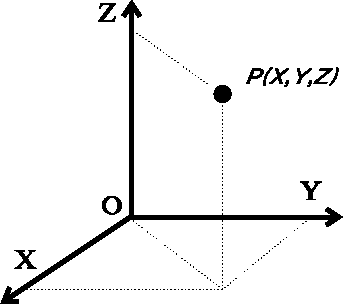Figure 6.6: Cartesian system of coordinates with orthogonal axes.

Cartesian coordinates are usually listed in 3-column format, X, Y, and Z coordinates for each atom. Sometime the coordinates are listed in natural crystal axes, called notional axes, which refer to the shape and dimensions of the unit cell. The notional axes are not generally perpendicular, and the coordinates are scaled by lengths of the unit cell edges. For the general case of a triclinic system, represented in Fig. 6.7, the edges of the unit cell along oblique axes, x, y and z, are a, b and c, respectively, and the interaxial angles:,and, are denoted byFigure 6.7: The unit cell with oblique axes for a triclinic crystallographic system,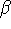and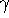, respectively. The coordinates expressed in such a system can be transformed to the orthogonal cartesian coordinates in several ways depending on the chosen orientation of the oblique system with respect to the cartesian system. One such formula, converting notional coordinates (x, y, z) to cartesian coordinates (x', y', z') is given below: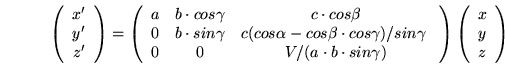(6.1)

NOTE: There was an error in the original text and the formula was given as: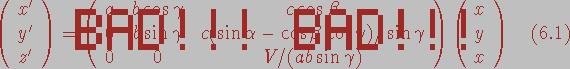.

Thanks to Egon Willighagen (egonw@sci.kun.nl) and Geoff Hutchison (hutchisn@chem.northwestern.edu) it was corrected on 2002.04.18.
where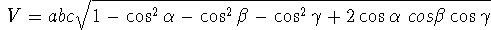Cartesian coordinates are an efficient representation of molecular geometry for the computer, and have the advantage of including actual spatial orientation of the molecule. However, they lack the chemical contents for chemists. Chemists prefer to specify and analyze molecular geometry in terms of internal coordinates, i.e., bond lengths and bond angles. The most popular internal coordinates are shown in Fig. 6.10, but before explaining how values of internal coordinates are calculated from cartesian coordinates of atoms, it is necessary to explain some of the simplest operations on vectors. The reader is encouraged to refer to the college calculus books for the review of vector analysis.Figure 6.8: Definition of vector in cartesian coordinate system.

A scalar quantity is just a number, e.g., molecular weight. A vector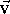can be imagined as an arrow starting at some point A and ending at some point B. It is important to realize that a vector is not in any way ``attached'' to points A and B, it merely represents a direction from point A to B and a distance between these points. If you translatedthe points to some other place, the vector between them would still remain the same. The vector is given by its 3 components, i.e., the lengths of its projections onto each of the three axes of the cartesian coordinate system (see Fig. 6.8),. The components,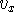,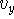,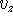are scalars, however, their sign depends on the direction of the vector. If the projection of the vector on the given axis points in the positive direction of the axis, the component is positive, otherwise, the component is negative. Two vectors are equal if their components are equal. If a vector is given by two points, its components are easily computed as differences between corresponding coordinates of the vector end (``head'') and the vector beginning (``tail''). In our case:The length of a vector is the distance between its beginning and its end. It is always positive (or zero, if the beginning and the end of a vector are in the same place). Formally, the vector length v (frequently written also as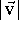) is given as the square root of the sum of the squares of its components: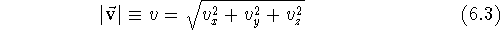As with scalars (i.e., ordinary numbers), certain operations are defined for vectors. Adding two vectors means forming a new vector whose components are the sums of the respective components of the vectors being added: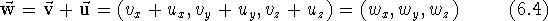Subtracting two vectors is analogous, only here the components are subtracted. You can multiply vector by a scalar by multiplying each of its components by the scalar: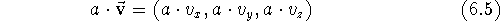Similarly, dividing a vector by a scalar results in a vector whose components are divided by this scalar, however, you obviously cannot divide by zero. Multiplying/dividing a vector by a scalar results in multiplying/dividing its length by this scalar, while preserving its direction. The unit vector is a vector whose length is equal to 1. You may obtain the unit vector from any vector by dividing it by its own length. Such an operation is called normalization of the vector and is usually denoted as: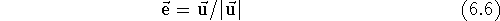Note that adding a scalar to a vector does not make any sense and is not among the defined operations.

There are two different modes for multiplying a vector by another vector. The scalar product of two vectors, also called the dot product, results in a scalar. It is the product of the vector lengths multiplied by the value of the cosine of the angle between them. It can also be calculated as the sum of the products of corresponding components:The dot product of two unit vectors is equal to the cosine of the angle between them. Hence, the cosine of an angle formed by two vectors is usually found by first normalizing the vectors and then calculating their dot product(by summing up the products of their components). Note also that the dot product does not depend upon the order in which the vectors are multiplied (i.e.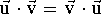).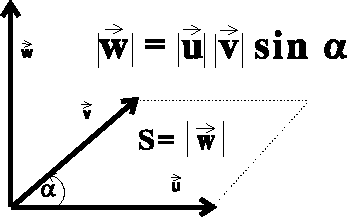Figure 6.9: Vector product of two vectors.

The vector product of two vectors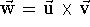, also called the cross product, results in a new vector. This vector is perpendicular to the plane in which the multiplied vectors lie, and points in the direction given by the right-hand screw rule (see Fig. 6.9). The order in which the vectors are multiplied is important, changing the order reverts the direction of the resulting vector. The length of the resulting vectoris equal in value to the area of the parallelogram constructed on the multilied vectors, i.e.: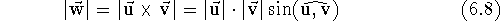Alternatively, the components of the resulting vectorare related to the components of vectorsandin the following way: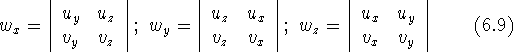where the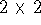determinant is defined as: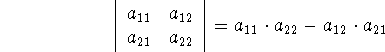You may frequently see the term base vectors, written as:,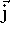and. These are unit vectors pointing along positive directions of x, y and z axes of the cartesian coordinate system. Note the simple relations between these vectors: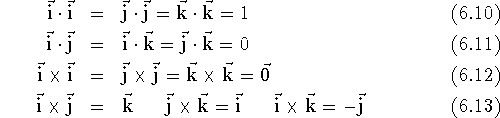and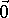denotes a zero vector, i.e., vector whose all components are zero.

Internal coordinates are efficiently calculated by the computer from cartesian coordinates using the vector operations described above. The bond length ,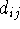(Fig. 6.10a), is simply a distance between two bonded atoms i and j, i.e., the length of the vector between atom i and j: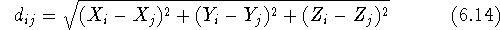The valence angle called also bond angle,(Fig. 6.10b), between bonds originating on atom j is calculated easily from the dot product of vectors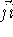and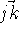. However, the cosine rule can also be used: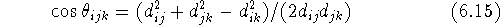The valence angle is always positive and not larger than 180, i.e., it is the smaller of the two possible angles.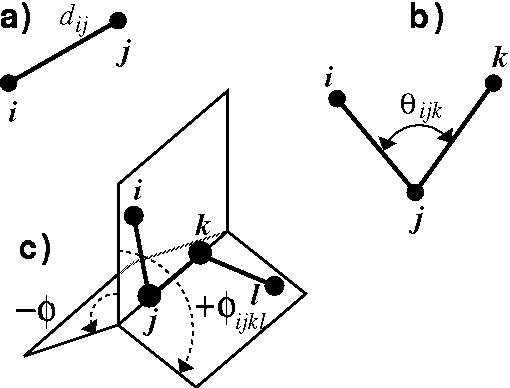Figure: Internal coordinates: a) bond length, b) valence angle, c) torsional angle.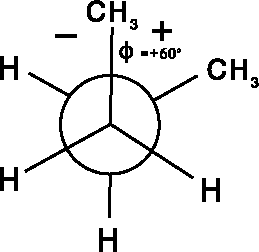Figure 6.11: Newman projection of the 60torsional angle for central C--C bond in butane.

The torsional angle (Fig. 6.10c), is a dihedral angle,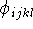, between two planes passing through atoms i,j,k and j,k,l, respectively. It is an angle between vectors normal (i.e., perpendicular) to these planes, or alternatively, an angle between the lines drawn on these planes perpendicularly to the edge where planes intersect. In contrast to the valence angle, the torsional angle spans the range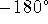to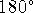, i.e., the full revolution. Its magnitude can be calculated as: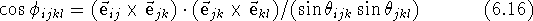whereis a unit vector pointing from atom i to j. Only the absolute value of the torsional angle can be derived by eq. 6.16. Additional checking has to be done to obtain the sign of the angle. Unfortunately two opposing conventions are used for the sign of a torsional angle. The chemists use the right-hand screw rule, as indicated by the arrows in Fig. 6.10c. In this case (assuming that we are looking along the direction j -- k and our eye is on the side of j) the clockwise turn from atom i to l, with j and k being the pivots, represents the positive angle while counterclockwise corresponds to negative values. Mathematicians, however, use an opposing rule in which the sign of the angle is positive for counterclockwise turns. Some modeling systems use this second convention and you should be aware of it. The torsional angles are frequently depicted as Newman projections as illustrated in Fig. 6.11 for butane.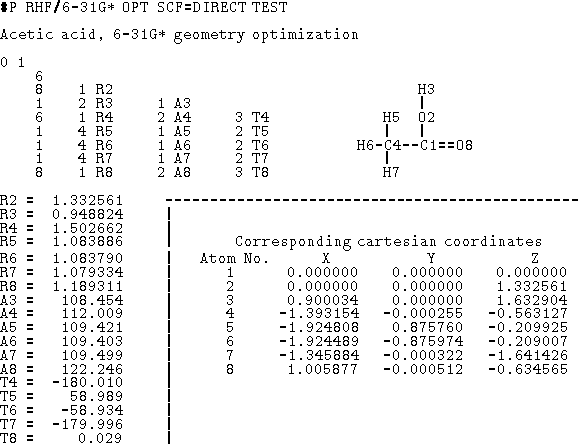Figure 6.12: Data for Gaussian 90 program in the form of a Z-matrix (cartesian coordinates included for reference). The first column of the Z-matrix corresponds to atomic number. The next columns represent atom numbers and internal coordinates (Rx -- distance, Ax -- valence angle, Tx -- torsional angle).

It is important to realize that the torsional angle is undefined if either atoms i, j and k, or j, k and l are collinear (i.e., a straight line is passing through three consecutive atoms) because an infinite number of planes can pass through three collinear points. For this reason, it does not make sense to talk about a torsional angle in acetylene.

An important property of torsional angles is that they do not depend upon the end from which they are measured, i.e.,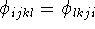. This stems from the same basic principle of geometry as the fact that a DNA strand is a right-handed helix irrespective from which terminus you look at it. You are encouraged to explore torsional angle properties with a bent paper clip or a folded piece of cardboard since this concept is essential for efficient work with any molecular modeling software system.

To fully specify molecular geometry to the computer in cartesian coordinates for a molecule containing N atoms, 3N values must be entered (i.e., X, Y and Z for each atom). The 3N coordinates specify not only intramolecular distances and angles but also the orientation of the molecule in space. Internal coordinates, on the other hand, specify only intramolecular distances and angles and the spatial orientation of the molecule is usually assumed. The popular way of specyfying molecular geometry using internal coordinates is a Z-matrix convention (see Fig. 6.12). Each line of the Z-matrix, with the exception of the first 3 lines, has the following format:

(i),, j,, k,l,where i is the number of the atom whose position is being defined. Since atoms are numbered consecutively, this number is equal to the current Z-matrix row number and this entry is often used for some mnemonic symbol for an atom or simply omitted as being redundant. The next entry,, is the atom type being defined (e.g., the atomic number), and j, k, and l refer to atoms whose positions were already defined in previous lines of the Z-matrix. The,andare: bond length, valence angle and torsional angle, respectively, formed by atom i with the corresponding atoms. In fact, in many cases,,andneed not be measured along chemical bonds but simply represent a purely geometrical relationship of atom i with previously defined atoms. The first three lines of the Z-matrix are shorter, because there is yet not enough defined atoms to specify distances and angles. The first line contains only the type of the first atom being defined,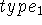. By convention, this atom is placed at the origin of the coordinate system. The second line, in addition to the atom type of the second atom, contains the distance from the first atom,. It is assumed by convention that bond 1--2 lies along the z-axis and points upwards towards the positive values of z. The third line contains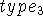as well as a distance d and an anglefor the third atom with respect to atoms 1 and 2. It is generally accepted that the third atom lies in the positive quadrant of the plane formed by the x and z axes. Some software packages adopt slightly different conventions for the Z-matrix (e.g., order of entries on the input line), incorporate a larger menu of internal coordinates (e.g., improper torsions, ring closures, etc.), and may contain some other information (e.g., atomic charges) together with the entries described above.

A Z-matrix requires only 3N-6 parameters (internal coordinates) for a full specification of molecular geometry (0 in first line, 1 in the second line, 2 in the third line, and 3(N-3) in the next N-3 lines). This is because the orientation of the molecule described by the Z-matrix is predefined, otherwise 6 additional parameters are needed to describe the orientation of a non-linear object in space (3 translational and 3 rotational degrees of freedom). In specifying internal geometry with a Z-matrix, dummy atoms are frequently used. Dummy atoms allow the specification of orientation other than the one dictated by the current convention for Z-matrix. They also must be used sometimes to account for collinear atoms in the molecule to avoid undefined torsional angles.

Cartesian coordinates are used as an input for many molecular modeling software systems. The particular format depends on the system being used. The most popular format used to describe the structure of a macromolecule is a PDBfile. A full description of the format of this file is available from Protein Data Bank at Brookhaven National Laboratory. All molecular modeling systems designed to work with biopolymers are capable of reading and producing files in this format. It is not well suited to represent small molecules, but on the other hand, no standard is generally accepted to describe the structure of small molecules. Therefore the PDB format needs be used sometimes as a vehicle to pass molecular structure information between software of different authors. A fragment of a PDB file is shown in Fig. 6.13. The file consists of records (lines), each 80 characters long. Each record (line) consists of a few fields. The last field at columns 71-80, contains the PDB file name and an ordinal number for the current record. The structure of records is fixed, i.e., each field starts at a prescribed column and has a strict format (e.g., number of decimal places). Some records have to follow in a strict order. Each record starts with a keyword which identifies the type of information in this record. Most keywords are self-explanatory and only few will be explained here.Figure 6.13: Fragment of a PDB file for an oligonucleotide.

• SEQRES -- one or more consecutively numbered records which list the sequence of residues for each chain of the macromolecule.
• HET -- identifies a non-standard group or residue. Consecutive entries denote: a nonstandard group identifier, a chain identifier if part of a chain, a sequence number in a chain, the number of atoms in a group, and explanatory text.
• FORMUL -- formula for a nonstandard group.
• CRYST -- unit cell definition: a, b and c in Å, and,andin degrees; and crystallographic space group.
• ATOM, HETATM -- the Cartesian coordinates of an atom in the standard (ATOM) and non-standard (HETATM) residue. Entries denote: atom serial number, atom name (each atom in the standard residue has its unique name assigned by the PDB standard), residue name, chain identifier, residue sequence number, cartesian coordinates X, Y, Z in Å, occupancy, the temperature factor.
• TER -- placed after the last atom in a chain. For proteins this is placed after the carboxy-terminal residue for each chain, and for nucleic acids it follows 3'-terminal residue of each strand.
• CONECT -- lists additional bonds (bonds within standard residues are usually not listed), like disulfide bridges. Hydrogen bonds, and salt bridges are also listed in these records. The first entry is the serial number of the atom being defined, followed by a list of atoms to which it is connected. Columns 12-31 are reserved for covalent bonds and columns 32-61 for hydrogen bonds and salt bridges.
• MASTER -- is a summary record, placed just before the end of the file, which contains a count of records for different types so the software can check the integrity of the file.
• END -- closes the PDB file.

In most cases the hydrogen atoms are not listed in the PDB file, since they are usually below the resolution of X-ray crystallography. Molecular modeling systems find the approximate positions of hydrogen atoms based on positions of heavy atoms, and therefore, PDB files processed by the modeling software may have these atoms appended.Next: Molecular surface and volume Up: Molecular Modeling Previous: Computer representation of chemical

Computational Chemistry
Wed Dec 4 17:47:07 EST 1996